## Example Questions

← Previous 1

### Example Question #1 : How To Find Circumference

If a circle has an area of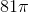, what is the circumference of the circle?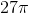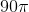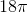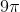Explanation:

The formula for  the area of a circle is πr2. For this particular circle, the area is 81π, so 81π = πr2. Divide both sides by π and we are left with r2=81. Take the square root of both sides to find r=9. The formula for the circumference of the circle is 2πr = 2π(9) = 18π. The correct answer is 18π.

### Example Question #3 : How To Find Circumference

A circle with an area of 13π in2 is centered at point C. What is the circumference of this circle?

√13π

26π

2√13π

13π

√26π

2√13π

Explanation:

The formula for the area of a circle is πr2.

We are given the area, and by substitution we know that 13π πr2.

We divide out the π and are left with 13 = r2.

We take the square root of r to find that r = √13.

We find the circumference of the circle with the formula = 2πr.

We then plug in our values to find = 2√13π.

### Example Question #4 : How To Find Circumference

A 6 by 8 rectangle is inscribed in a circle. What is the circumference of the circle?

8π

6π

25π

10π

12π

10π

Explanation:

First you must draw the diagram. The diagonal of the rectangle is also the diameter of the circle. The diagonal is the hypotenuse of a multiple of 2 of a 3,4,5 triangle, and therefore is 10.
Circumference = π * diameter = 10π.

### Example Question #1 : How To Find Circumference

A gardener wants to build a fence around their garden shown below. How many feet of fencing will they need, if the length of the rectangular side is 12 and the width is 8?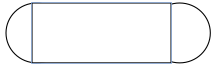40 ft.

96 ft

8π + 24

4π + 24

8π + 24

Explanation:

The shape of the garden consists of a rectangle and two semi-circles. Since they are building a fence we need to find the perimeter. The perimeter of the length of the rectangle is 24. The perimeter or circumference of the circle can be found using the equation C=2π(r), where r= the radius of the circle. Since we have two semi-circles we can find the circumference of one whole circle with a radius of 4, which would be 8π.

### Example Question #6 : How To Find Circumference

The diameter of a circle is defined by the two points (2,5) and (4,6). What is the circumference of this circle?

2.5π

π√2.5

π√5

π√5

Explanation:

We first must calculate the distance between these two points. Recall that the distance formula is:√((x2 - x1)2 + (y2 - y1)2)

For us, it is therefore: √((4 - 2)2 + (6 - 5)2) = √((2)2 + (1)2) = √(4 + 1) = √5

If d = √5, the circumference of our circle is πd, or π√5.

### Example Question #5 : How To Find Circumference

A car tire has a radius of 18 inches. When the tire has made 200 revolutions, how far has the car gone in feet?

3600π

600π

300π

500π

600π

Explanation:

If the radius is 18 inches, the diameter is 3 feet. The circumference of the tire is therefore 3π by C=d(π). After 200 revolutions, the tire and car have gone 3π x 200 = 600π feet.

### Example Question #1 : How To Find Circumference

A circle has the equation below. What is the circumference of the circle?

(x – 2)2 + (y + 3)2 = 9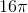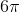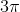Explanation:

The radius is 3. Yielding a circumference of.

### Example Question #1 : How To Find Circumference

Find the circumferencce fo a circle given radius of 7.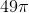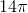Explanation:

To solve, simply use the formula for the circumference of a circle. Thus,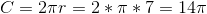Like the prior question, it is important to think about dimensions if you don't remember the exact formula. Circumference is 1 dimensional, so it makes sense that the variable is not squared as cubed. If you rather, you can use the following formula, but realize by defining diameter, it equals the prior one.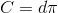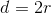Thus,### Example Question #2 : How To Find Circumference

The area of a circle is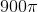. What is its circumference?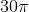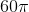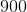Explanation:

First, let's find the radius r of the circle by using the given area.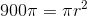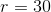Now, plug this radius into the formula for a circle's circumference.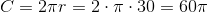### Example Question #3 : How To Find Circumference

The surface area of a sphere is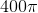.is a point on the surface of the sphere;is the point on the sphere farthest from. A curve is drawn fromtoentirely on the surface of the sphere. Give the length of the shortest possible curve fitting this description.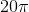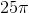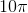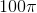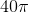Explanation:

Below is a sphere with its center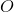and with pointsandas described.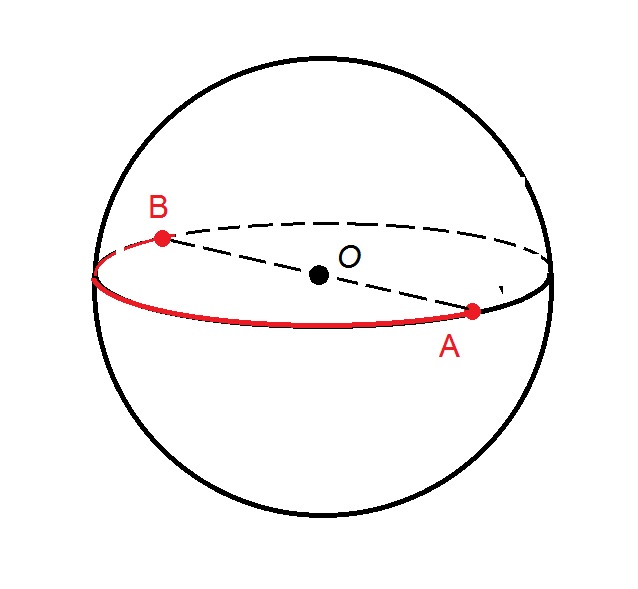Forandto be on the surface of the sphere and to be a maximum distance apart, they must be endpoints of a diameter of the sphere. The shortest curve connecting them that is entirely on the surface is a semicircle whose radius coincides with that of the sphere. Therefore, first find the radius of the sphere using the surface area formula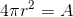Setting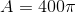and solving for: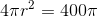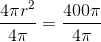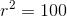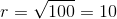The length of the curve is half the circumference of a circle with radius 10, orSubstituting 10 for, this is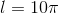.

← Previous 1